# GBDT算法原理以及实例理解

【尊重原创，转载请注明出处】http://blog.csdn.net/zpalyq110/article/details/79527653

Github：https://github.com/Freemanzxp/GBDT_Simple_Tutorial

## 1. Decision Tree：CART回归树

首先，GBDT使用的决策树是CART回归树，无论是处理回归问题还是二分类以及多分类，GBDT使用的决策树通通都是都是CART回归树。为什么不用CART分类树呢？因为GBDT每次迭代要拟合的是梯度值，是连续值所以要用回归树。
对于回归树算法来说最重要的是寻找最佳的划分点，那么回归树中的可划分点包含了所有特征的所有可取的值。在分类树中最佳划分点的判别标准是熵或者基尼系数，都是用纯度来衡量的，但是在回归树中的样本标签是连续数值，所以再使用熵之类的指标不再合适，取而代之的是平方误差，它能很好的评判拟合程度。

（1）选择最优切分变量$j$与切分点$s$，求解
$\underset{j,s}{min}\left [ \underset{c_1}{min}\underset{x_i\in R_1(j,s)}{\sum}(y_i-c_1)^2 + \underset{c_2}{min}\underset{x_i\in R_2(j,s)}{\sum}(y_i-c_2)^2\ \right ]$遍历变量$j$，对固定的切分变量$j$扫描切分点$s$，选择使得上式达到最小值的对$(j,s)$.
（2）用选定的对$(j,s)$划分区域并决定相应的输出值：$R_1(j,s)={x|x^{(j)}\leq s}, R_2(j,s)={x|x^{(j)}> s}$$\hat{c_m} =\frac{1}{N}\underset{x_1\in R_m(j,s)}{\sum}y_i, x \in R_m, m=1,2$（3）继续对两个子区域调用步骤（1）和（2），直至满足停止条件。
（4）将输入空间划分为$M$个区域 $R_1,R_2,...R_M$，生成决策树：
$f(x)=\sum_{m=1}^{M}\hat{c}_m I(x \in R_m)$

梯度提升树（Grandient Boosting）是提升树（Boosting Tree）的一种改进算法，所以在讲梯度提升树之前先来说一下提升树。

先来个通俗理解：假如有个人30岁，我们首先用20岁去拟合，发现损失有10岁，这时我们用6岁去拟合剩下的损失，发现差距还有4岁，第三轮我们用3岁拟合剩下的差距，差距就只有一岁了。如果我们的迭代轮数还没有完，可以继续迭代下面，每一轮迭代，拟合的岁数误差都会减小。最后将每次拟合的岁数加起来便是模型输出的结果。

（1）初始化$f_0(x)=0$
（2）对$m=1,2,...,M$
（a）计算残差$r_{mi}=y_i-f_{m-1}(x), i=1,2,...,N$ （b）拟合残差$r_{mi}$学习一个回归树，得到$h_m(x)$
（c）更新$f_m(x) = f_{m-1}+h_m(x)$
（3）得到回归问题提升树$f_M(x)=\sum_{m=1}^Mh_m(x)$

回到我们上面讲的那个通俗易懂的例子中，第一次迭代的残差是10岁，第二 次残差4岁……

当损失函数是平方损失和指数损失函数时，梯度提升树每一步优化是很简单的，但是对于一般损失函数而言，往往每一步优化起来不那么容易，针对这一问题，Friedman提出了梯度提升树算法，这是利用最速下降的近似方法，其关键是利用损失函数的负梯度作为提升树算法中的残差的近似值。

那么对于分类问题呢？二分类和多分类的损失函数都是$log loss$本文以回归问题为例进行讲解

## 3. GBDT算法原理

GBDT算法：
（1）初始化弱学习器
$f_0(x) = {arg\;min}_{c}\sum\limits_{i=1}^{N}L(y_i, c)$（2）对$m=1,2,...,M$有：
（a）对每个样本$i=1,2,...,N$，计算负梯度，即残差
$r_{im} = -\bigg[\frac{\partial L(y_i, f(x_i)))}{\partial f(x_i)}\bigg]_{f(x) = f_{m-1} (x)}$
（b）将上步得到的残差作为样本新的真实值，并将数据$(x_i,r_{im})， i=1,2,..N$作为下棵树的训练数据，得到一颗新的回归树$f_{m} (x)$其对应的叶子节点区域为$R_{jm}, j =1,2,..., J$。其中$J$为回归树t的叶子节点的个数。
（c）对叶子区域$j =1,2,..J$计算最佳拟合值
$\Upsilon_{jm} = \underbrace{arg\; min}_{\Upsilon}\sum\limits_{x_i \in R_{jm}} L(y_i,f_{m-1}(x_i) +\Upsilon)$ （d）更新强学习器
$f_{m}(x) = f_{m-1}(x) + \sum\limits_{j=1}^{J}\Upsilon_{jm}I(x \in R_{jm})$（3）得到最终学习器
$f(x) = f_M(x) =f_0(x) + \sum\limits_{m=1}^{M}\sum\limits_{j=1}^{J}\Upsilon_{jm}I(x \in R_{jm})$

## 4. 实例详解

Github：https://github.com/Freemanzxp/GBDT_Simple_Tutorial

### 数据介绍：

如下表所示：一组数据，特征为年龄、体重，身高为标签值。共有5条数据，前四条为训练样本，最后一条为要预测的样本。

0 5 20 1.1
1 7 30 1.3
2 21 70 1.7
3 30 60 1.8
4(要预测的) 25 65

### 训练阶段：

• 学习率：learning_rate=0.1
• 迭代次数：n_trees=5
• 树的深度：max_depth=3

1.初始化弱学习器:$f_0(x) = {arg\;min}_{c}\sum\limits_{i=1}^{N}L(y_i, c)$  损失函数为平方损失，因为平方损失函数是一个凸函数，直接求导，倒数等于零，得到$c$
$\sum\limits_{i=1}^{N}\frac{\partial L(y_i,c))}{\partial c}=\sum\limits_{i=1}^{N}\frac{\partial (\frac{1}{2}(y_i-c)^2)}{\partial c}=\sum\limits_{i=1}^{N}c-y_i$令导数等于0
$\sum\limits_{i=1}^{N}c-y_i=0$$c=(\sum\limits_{i=1}^{N}y_i)/N$所以初始化时，$c$取值为所有训练样本标签值的均值。$c=(1.1+1.3+1.7+1.8)/4=1.475$，此时得到初始学习器$f_0(x)$
$f_0(x) = c=1.475$

2.对迭代轮数m=1，2,…,M:
由于我们设置了迭代次数：n_trees=5，这里的$M=5$
计算负梯度，根据上文损失函数为平方损失时，负梯度就是残差残差，再直白一点就是 $y$与上一轮得到的学习器$f_{m-1}$的差值
$r_{i1} = -\bigg[\frac{\partial L(y_i, f(x_i)))}{\partial f(x_i)}\bigg]_{f(x) = f_{0} (x)}$

0 1.1 1.475 -0.375
1 1.3 1.475 -0.175
2 1.7 1.475 0.225
3 1.8 1.475 0.325

0 5 20 -0.375
1 7 30 -0.175
2 21 70 0.225
3 30 60 0.325

接着，寻找回归树的最佳划分节点，遍历每个特征的每个可能取值。从年龄特征的5开始，到体重特征的70结束，分别计算分裂后两组数据的平方损失（Square Error），$SE_l$左节点平方损失，$SE_r$右节点平方损失，找到使平方损失和$SE_{sum}=SE_l+SE_r$最小的那个划分节点，即为最佳划分节点。
例如：以年龄7为划分节点，将小于7的样本划分为到左节点，大于等于7的样本划分为右节点。左节点包括$x_0$，右节点包括样本$x_1,x_2,x_3$$SE_l = 0,SE_r=0.140,SE_{sum}=0.140$，所有可能划分情况如下表所示：

以上划分点是的总平方损失最小为0.025有两个划分点：年龄21和体重60，所以随机选一个作为划分点，这里我们选 年龄21
现在我们的第一棵树长这个样子：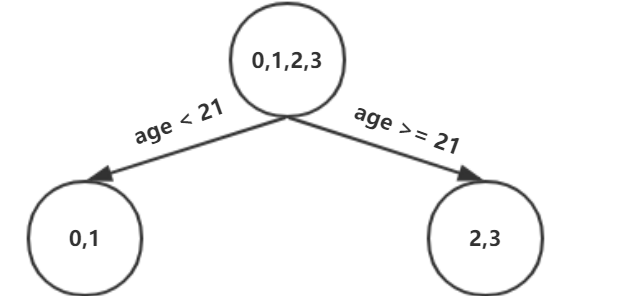我们设置的参数中树的深度max_depth=3，现在树的深度只有2，需要再进行一次划分，这次划分要对左右两个节点分别进行划分：

对于左节点，只含有0,1两个样本，根据下表我们选择年龄7划分

对于右节点，只含有2,3两个样本，根据下表我们选择年龄30划分（也可以选体重70

现在我们的第一棵树长这个样子：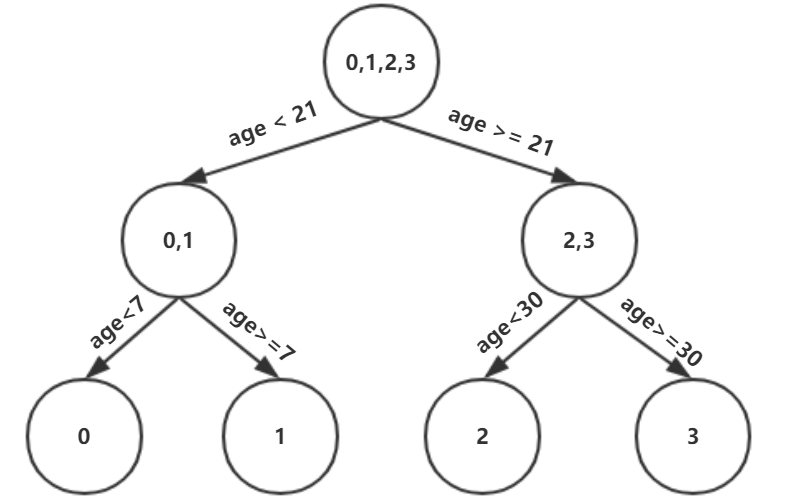此时我们的树深度满足了设置，还需要做一件事情，给这每个叶子节点分别赋一个参数$\Upsilon$，来拟合残差。$\Upsilon_{j1} = \underbrace{arg\; min}_{\Upsilon}\sum\limits_{x_i \in R_{j1}} L(y_i,f_{0}(x_i) +\Upsilon)$  这里其实和上面初始化学习器是一个道理，平方损失，求导，令导数等于零，化简之后得到每个叶子节点的参数$\Upsilon$，其实就是标签值的均值。这个地方的标签值不是原始的 $y$，而是本轮要拟合的标残差 $y-f_0(x)$.
根据上述划分结果，为了方便表示，规定从左到右为第$1,2,3,4$个叶子结点$(x_0 \in R_{11})，\Upsilon_{11}=-0.375$$(x_1 \in R_{21})，\Upsilon_{21}=-0.175$$(x_2 \in R_{31})，\Upsilon_{31}=0.225$$(x_3 \in R_{41})，\Upsilon_{41}=0.325$
此时的树长这个样子：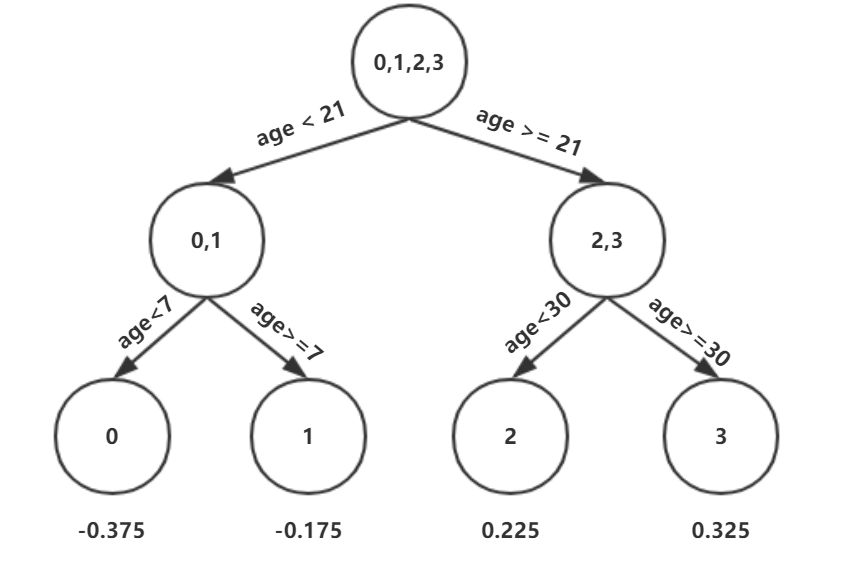此时可更新强学习器，需要用到参数学习率：learning_rate=0.1，用$lr$表示。
$f_{1}(x) = f_{0}(x) + lr *\sum\limits_{j=1}^{4}\Upsilon_{j1}I(x \in R_{j1})$

Github：https://github.com/Freemanzxp/GBDT_Simple_Tutorial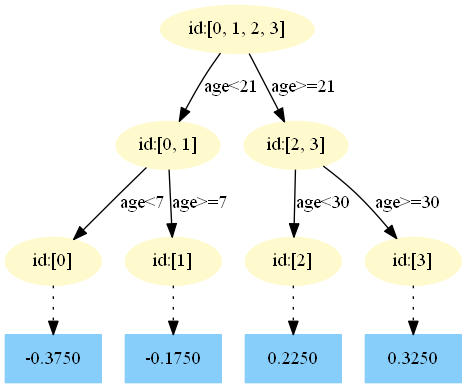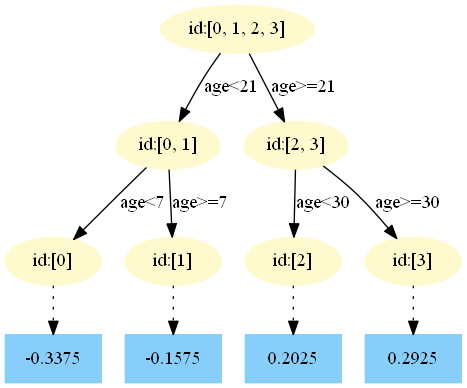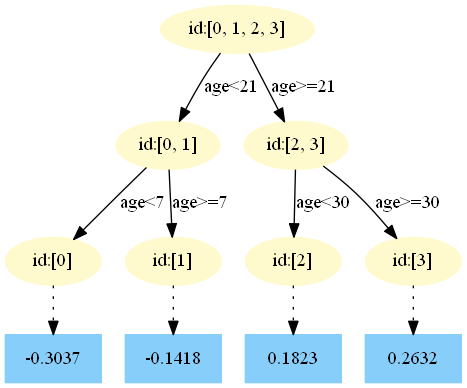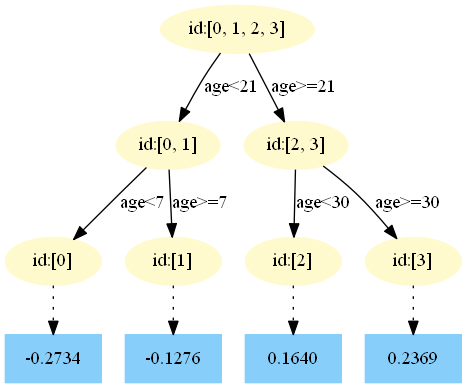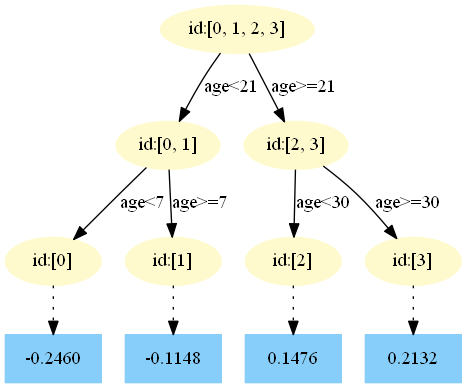4.得到最后的强学习器：
$f(x) = f_{5}(x) =f_0(x) + \sum\limits_{m=1}^{5}\sum\limits_{j=1}^{4}\Upsilon_{jm}I(x \in R_{jm})$

5.预测样本5：
$f_0(x)=1.475$
在$f_1(x)$中，样本4的年龄为25，大于划分节点21岁，又小于30岁，所以被预测为0.2250
在$f_2(x)$中，样本4的…此处省略…所以被预测为0.2025
为什么是0.2025？这是根据第二颗树得到的，可以GitHub简单运行一下代码
在$f_3(x)$中，样本4的…此处省略…所以被预测为0.1823
在$f_4(x)$中，样本4的…此处省略…所以被预测为0.1640
在$f_5(x)$中，样本4的…此处省略…所以被预测为0.1476

## 参考资料

1. 李航 《统计学习方法》
2. Friedman J H . Greedy Function Approximation: A Gradient Boosting Machine[J]. The Annals of Statistics, 2001, 29(5):1189-1232.

【尊重原创，转载请注明出处】 http://blog.csdn.net/zpalyq110/article/details/79527653

©️2019 CSDN 皮肤主题: 编程工作室 设计师: CSDN官方博客